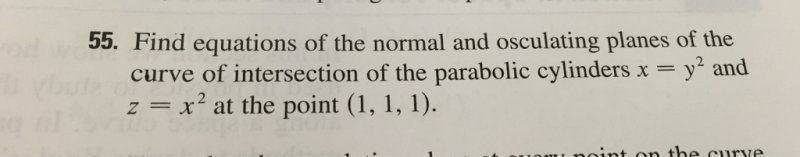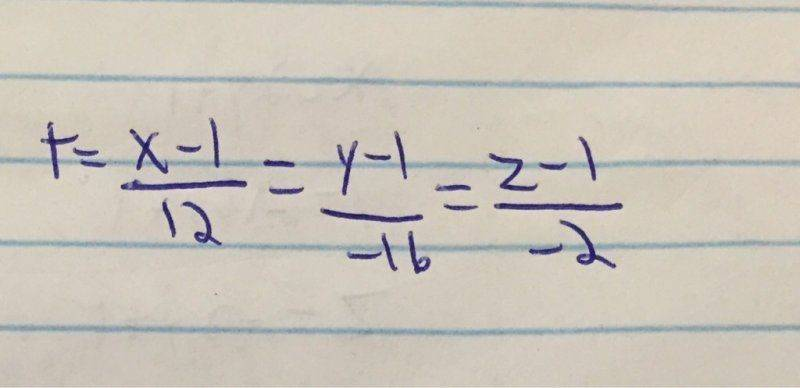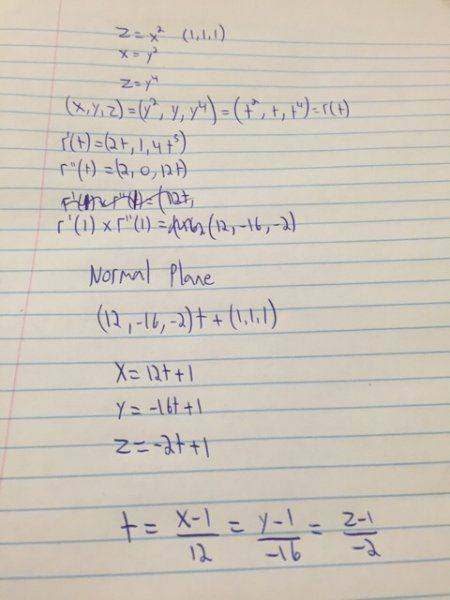# How to turn these symmetric equations into the general form?

• Regtic
In summary, the student tried to solve a problem using a method that wasn't the recommended way and was having trouble. The student was told that the problem wasn't solved using that method and was shown the correct way. The problem was solved using a different method that the student had forgotten.

#### Regtic

I was solving this problemand I didn't want to do it the really long way by finding the equation of B(t) by first finding T(t) and N(t). So i took the cross product of r' and r'' so that they would be in the direction of B. Found the parametric equation of the plane but the book answer was in the general format.How do I turn this into the general format to check my answer? It should be 2X +Y +4Z -7 = 0

Whenever I try i just get two equations that don't mix to give me x y and z in one equation but it should be right.

Last edited:
Regtic said:
I was solving this problem View attachment 76531

and I didn't want to do it the really long way by finding the equation of B(t) by first finding T(t) and N(t). So i took the cross product of r' and r'' and took their cross product so that they would be in the direction of B. Found the parametric equation of the plane but the book answer was in the general format. View attachment 76532

How do I turn this into the general format to check my answer? It should be 2X +Y +4Z -7 = 0

Whenever I try i just get two equations that don't mix to give me x y and z in one equation but it should be right.

That's not the equation of a plane. It's the equation of a line. You might want to try the long way.

Why can't you do it my way? I know it works, I've done it on other problems. My teacher's done it like that before too.

Regtic said:
Why can't you do it my way? I know it works, I've done it on other problems. My teacher's done it like that before too.

I don't know what you are doing. Can you show us?

Redid it to make it a bit clearerI'm with Dick here. The symmetric equations you show in post #1 are for a line, not a plane.

In your 2nd attachment, under "Normal Plane" you have (12, -16, -2)t (1, 1, 1). That can't be the equation of a plane, because (1) it's not an equation, and (2) there should be two parameters, not just one, in the parametric form of the equation of a plane.

Mark44 said:
In your 2nd attachment, under "Normal Plane" you have (12, -16, -2)t (1, 1, 1). That can't be the equation of a plane, because (1) it's not an equation, and (2) there should be two parameters, not just one, in the parametric form of the equation of a plane.
Ooo right. Damn, I forgot a lot from linear. Literally not a plane with only 1 parameter. Ok thanks